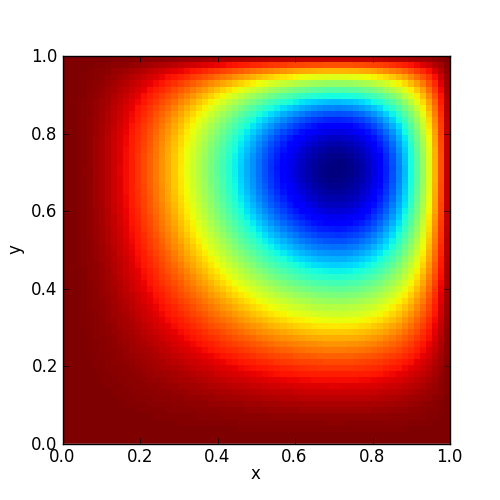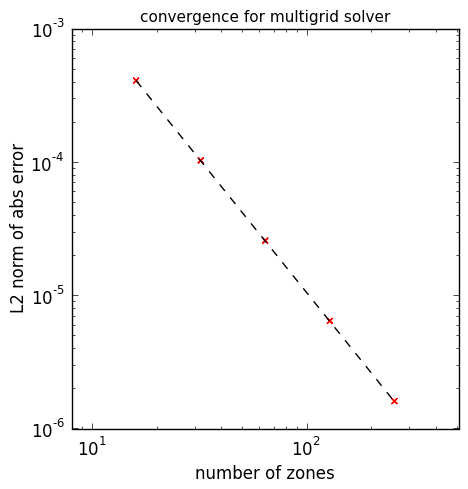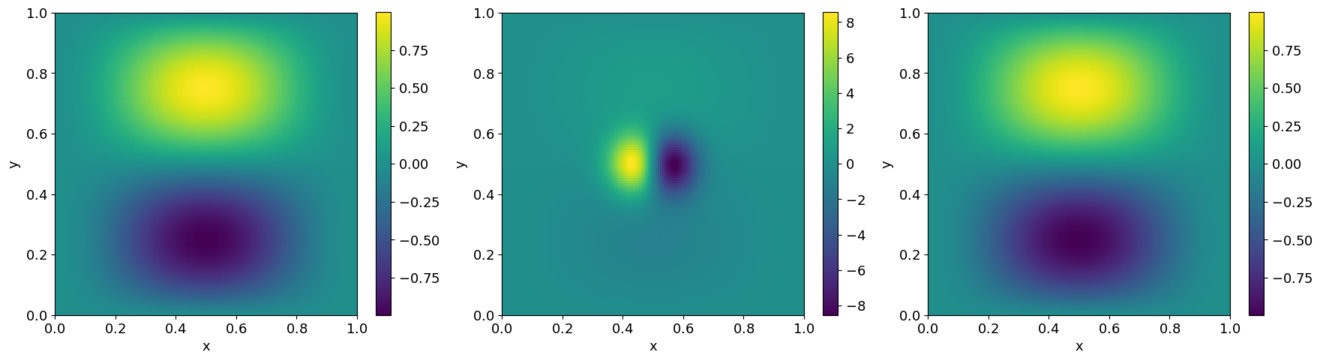# Multigrid solvers¶

pyro solves elliptic problems (like Laplace’s equation or Poisson’s equation) through multigrid. This accelerates the convergence of simple relaxation by moving the solution down and up through a series of grids. Chapter 9 of the pdf notes gives an introduction to solving elliptic equations, including multigrid.

There are three solvers:

• The core solver, provided in the class MG.CellCenterMG2d solves constant-coefficient Helmholtz problems of the form $$(\alpha - \beta \nabla^2) \phi = f$$

• The class variable_coeff_MG.VarCoeffCCMG2d solves variable coefficient Poisson problems of the form $$\nabla \cdot (\eta \nabla \phi ) = f$$. This class inherits the core functionality from MG.CellCenterMG2d.

• The class general_MG.GeneralMG2d solves a general elliptic equation of the form $$\alpha \phi + \nabla \cdot ( \beta \nabla \phi) + \gamma \cdot \nabla \phi = f$$. This class inherits the core functionality from MG.CellCenterMG2d.

This solver is the only one to support inhomogeneous boundary conditions.

We simply use V-cycles in our implementation, and restrict ourselves to square grids with zoning a power of 2.

The multigrid solver is not controlled through pyro.py since there is no time-dependence in pure elliptic problems. Instead, there are a few scripts in the multigrid/ subdirectory that demonstrate its use.

## Examples¶

### multigrid test¶

A basic multigrid test is run as (using a path relative to the root of the pyro2 repository):

./examples/multigrid/mg_test_simple.py


The mg_test_simple.py script solves a Poisson equation with a known analytic solution. This particular example comes from the text A Multigrid Tutorial, 2nd Ed., by Briggs. The example is:

$u_{xx} + u_{yy} = -2 \left [(1-6x^2)y^2(1-y^2) + (1-6y^2)x^2(1-x^2)\right ]$

on $$[0,1] \times [0,1]$$ with $$u = 0$$ on the boundary.

The solution to this is shown below.Since this has a known analytic solution:

$u(x,y) = (x^2 - x^4)(y^4 - y^2)$

We can assess the convergence of our solver by running at a variety of resolutions and computing the norm of the error with respect to the analytic solution. This is shown below:The dotted line is 2nd order convergence, which we match perfectly.

The movie below shows the smoothing at each level to realize this solution:

You can run this example locally by running the mg_vis.py script:

./examples/multigrid/mg_vis.py


### projection¶

Another example uses multigrid to extract the divergence free part of a velocity field. This is run as:

./examples/multigrid/project_periodic.py


Given a vector field, $$U$$, we can decompose it into a divergence free part, $$U_d$$, and the gradient of a scalar, $$\phi$$:

$U = U_d + \nabla \phi$

We can project out the divergence free part by taking the divergence, leading to an elliptic equation:

$\nabla^2 \phi = \nabla \cdot U$

The project-periodic.py script starts with a divergence free velocity field, adds to it the gradient of a scalar, and then projects it to recover the divergence free part. The error can found by comparing the original velocity field to the recovered field. The results are shown below:Left is the original u velocity, middle is the modified field after adding the gradient of the scalar, and right is the recovered field.

## Exercises¶

### Explorations¶

• Try doing just smoothing, no multigrid. Show that it still converges second order if you use enough iterations, but that the amount of time needed to get a solution is much greater.

### Extensions¶

• Implement inhomogeneous dirichlet boundary conditions

• Add a different bottom solver to the multigrid algorithm

• Make the multigrid solver work for non-square domains